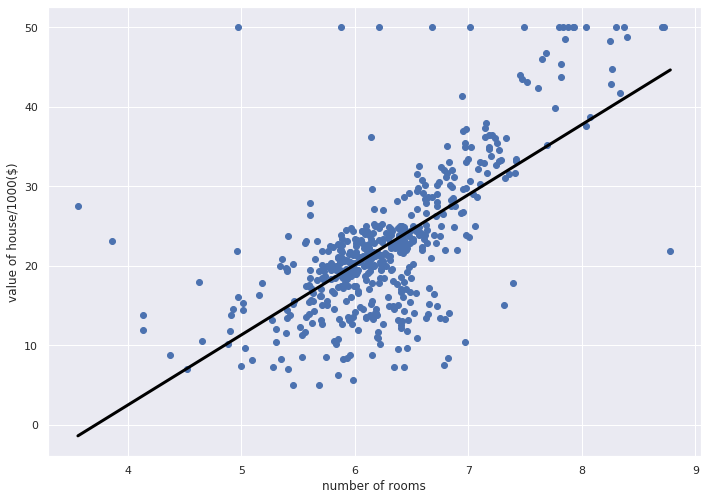Search

# Linear Regression: Boston Housing data setWe will work with Boston housing data set which consists information about houses in Boston. It is provided in scikit-learn library. There are 506 samples and 13 feature variables in this dataset. The objective is to predict the value of prices of the house based on number of rooms. For this we will implement linear regression.

First we will load the Boston data set from sklearn.datasets and then we will convert it into a dataframe using pandas so that we can easily work with it. We use NumPy to work with arrays.We will use matplotlib and seaborn to visualise the data.

```import numpy as np
import pandas as pd
import seaborn as sns
import matplotlib.pyplot as plt
%matplotlib inline
from sklearn import datasets
from sklearn.linear_model import LinearRegression
from sklearn.model_selection import train_test_split, cross_val_score
from sklearn.metrics import mean_squared_error
boston.keys()```

Output: dict_keys(['data', 'target', 'feature_names', 'DESCR', 'filename'])

data: contains the information for various houses

target: prices of the house

feature_names: names of the features

DESCR: describes the data set

```bos = pd.DataFrame(boston.data, columns = boston.feature_names)
bos['PRICE'] = boston.targetNow, we will explore the data to understand it better.

We will look at the descriptive statistics of the data using the describe( ) function.

`bos.describe()`We will plot a histogram of PRICE feature.

```sns.set(rc={'figure.figsize':(11.7,8.27)})
plt.hist(bos['PRICE'],color = 'red', bins=30)
plt.xlabel("House prices in \$1000")
plt.show()```We observe that the data is distributed normally and that there are only a few outliers.

Next, we create a correlation matrix that measures the linear relationships between the variables. It can be done by using the function corr( ).

We will use the heatmap function from the seaborn library to plot the correlation matrix.

```bos_1 = pd.DataFrame(boston.data, columns = boston.feature_names)
bos_1['PRICE']=boston.target
correlation_matrix = bos_1.corr().round(2)
sns.heatmap(data=correlation_matrix, annot=True)```The correlation coefficient ranges from -1 to 1. If the value is close to 1, it means that there is a strong positive correlation between the two variables. If it is close to -1, the variables have a strong negative correlation.

We prefer to use features with high correlation (whether positive or negative) with our target feature PRICE, to fit to the model. The feature RM has a strong positive correlation with PRICE (0.7) where as LSTAT has a high negative correlation (-0.74).

We will draw a scatterplot of RM and LSTAT against PRICE to better visualise the correlation.

```plt.figure(figsize=(20, 5))

features = ['LSTAT', 'RM']
target = bos['PRICE']

for i, col in enumerate(features):
plt.subplot(1, len(features) , i+1)
x = bos[col]
y = target
plt.scatter(x, y,color='green', marker='o')
plt.title("Variation in House prices")
plt.xlabel(col)
plt.ylabel('"House prices in \$1000"')```Also, an important point in selecting features for a linear regression model is to check for multi-co-linearity. The features RAD, TAX have a correlation of 0.91. These feature pairs are strongly correlated to each other. We should not select both these features together for training the model. Same goes for the features DIS and AGE which have a correlation of -0.75.

Since we want to predict prices based on number of rooms only. We will only use RM feature to train on the model and PRICE feature will be our target.

```X_rooms = bos.RM
y_price = bos.PRICE

X_rooms = np.array(X_rooms).reshape(-1,1)
y_price = np.array(y_price).reshape(-1,1)
X_train_1, X_test_1, Y_train_1, Y_test_1 = train_test_split(X_rooms, y_price, test_size = 0.2, random_state=5)```

We have split the data set into training and test set. So, that we can make sure that our model performs well on unseen data.

We fit our training data to LinearRegresion model and train it. We also calculate the RMSE (root mean square error) and R2 score to see how well our model performs.

```reg_1 = LinearRegression()
reg_1.fit(X_train_1, Y_train_1)

y_train_predict_1 = reg_1.predict(X_train_1)
rmse = (np.sqrt(mean_squared_error(Y_train_1, y_train_predict_1)))
r2 = round(reg_1.score(X_train_1, Y_train_1),2)

print('RMSE is {}'.format(rmse))
print('R2 score is {}'.format(r2))
print("\n")```

We get : RMSE is 6.972277149440585 R2 score is 0.43

Our model has been trained. Now, we will use it on test data.

```reg_1 = LinearRegression()
reg_1.fit(X_train_1, Y_train_1)

y_train_predict_1 = reg_1.predict(X_train_1)
rmse = (np.sqrt(mean_squared_error(Y_train_1, y_train_predict_1)))
r2 = round(reg_1.score(X_train_1, Y_train_1),2)

print('RMSE is {}'.format(rmse))
print('R2 score is {}'.format(r2))
print("\n")

```

We get: Root Mean Squared Error: 4.895963186952216 R^2: 0.69

We can see that our model worked better on the test set.

We will now plot or predictions:

```prediction_space = np.linspace(min(X_rooms), max(X_rooms)).reshape(-1,1)
plt.scatter(X_rooms,y_price)
plt.plot(prediction_space, reg_1.predict(prediction_space), color = 'black', linewidth = 3)
plt.ylabel('value of house/1000(\$)')
plt.xlabel('number of rooms')
plt.show()```### Recent Posts

See All

#### Deploying Machine Learning Models in SageMaker - AWS Cloud.

Tel: (+91) 0120  4118730

Time :   10 : 00  AM -  08 : 00 PM IST